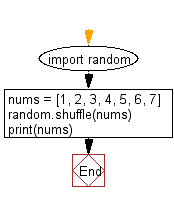﻿ Python Math: Shuffle the following elements randomly - w3resource# Python Math: Shuffle the following elements randomly

## Python Math: Exercise-52 with Solution

Write a Python program to shuffle the following elements randomly.

Sample Solution:-

Python Code:

``````import random

nums = [1, 2, 3, 4, 5, 6, 7]
random.shuffle(nums)
print(nums)
```
```

Sample Output:

```[5, 3, 4, 7, 1, 6, 2]
```

Pictorial Presentation:Flowchart:## Visualize Python code execution:

The following tool visualize what the computer is doing step-by-step as it executes the said program:

Python Code Editor:

Have another way to solve this solution? Contribute your code (and comments) through Disqus.

What is the difficulty level of this exercise?

Test your Programming skills with w3resource's quiz.

﻿

```>>> students = [{'name': 'John', 'score': 98}, {'name': 'Mike', 'score': 94}, {'name': 'Jennifer', 'score': 99}]